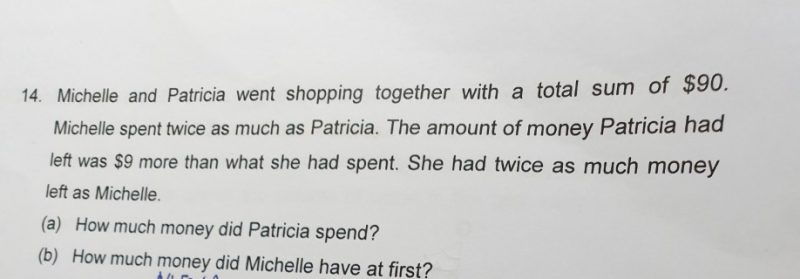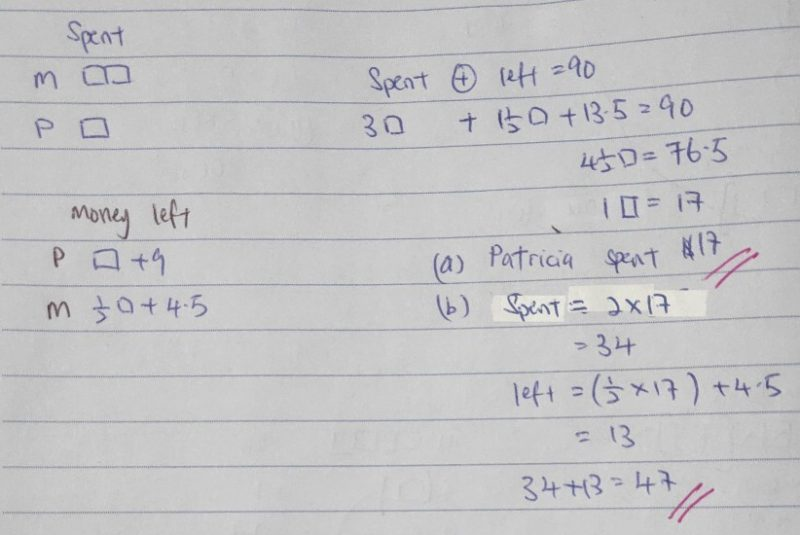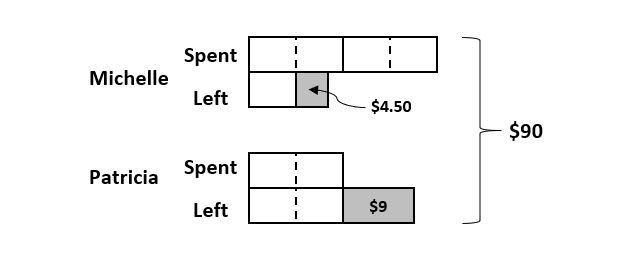QuestionDear teachers pls teach me how to solve this queation. ThanksWebsite

0 Replies 0 Likes
 Michelle Patricia Spent 4U 2U Left 1U + 4.5 2U + 9 Had 5U + 4.5 4U + 9

5U + 4.5 + 4U + 9 = 90, 9U + 13.5 = 90, 9U = 76.5, 1U = 8.5\$

(a) Patricia spent 2U. 2U = 2 x 8.5 = \$17

(b) Michelle had = 5U + 4.5 = 5 x 8.5 + 4.5 = 47\$

Verify

 Michelle Patricia Had 47\$ 43\$ Spent -34\$ -17\$ Left with 13\$ 26\$

13 x 2 = 26\$

0 Replies 0 Likes9 units = \$90 – \$9 – \$4.50 = \$76.50

1 unit = \$76.50 ÷ 9 = \$8.50

2 units = 2 x \$8.50 = \$17

Answer: (a) Patricia spent \$17.

5 units = 5 x \$8.50 = \$42.50

\$42.50 + \$4.50 = \$47

Answer: (b) Michelle had \$47 at first.

0 Replies 0 Likes